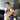# NSObject Equality is Tricky

November 13, 2016

Update (4/27/2020): 4 years after writing this article, I realized that it could be improved on a little bit. Added info about the recommended way to override equality for NSObjects, and a note about how Swiftlint has a rule to handle this.

Swift can be tricky sometimes. For example, what does the following print?

``````class A: NSObject {
let x: Int

init(x: Int) {
self.x = x
}
}

func ==(left: A, right: A) -> Bool {
return left.x == right.x
}

print(A(x: 1) == A(x: 1))
print([A(x: 1)] == [A(x: 1)])``````

Perhaps surprisingly, it's this:

``````true
false // huh?!``````

### Equatable conformance is hard

Why does comparing two arrays with equal contents return false? It turns out that:

• Array is bridged to NSArray
• NSArray doesn't declare a `==(_:_:)` function, so it falls back to NSObject's `==(_:_:)`, which calls `isEqual`
• NSArray's `isEqual` compares objects by casting to AnyHashable

We can see that this is the problem by casting the objects to `AnyHashable` before comparing them:

``print(A(x: 1) as AnyHashable == A(x: 1) as AnyHashable)``
``false``

Apparently, doing this will use the NSObject implementation of `==(_:_:)` rather than the `A` one. The NSObject implementation of `==(_:_:)` checks isEqual, which returns false because the two elements aren't the same in memory. We can see this by adding an override:

``````class B: NSObject {
let x: Int

init(x: Int) {
self.x = x
}

override func isEqual(_ object: Any?) -> Bool {
print("isEqual for B")
return super.isEqual(object)
}
}

func ==(left: B, right: B) -> Bool {
print("== for B")
return left.x == right.x
}``````

Then,

``print([B(x: 1)] == [B(x: 1)])``
``````isEqual for B
false``````

### The fix

The best way to make an NSObject subclass use custom equality inside an array is to override isEqual:

``````class C: NSObject {
let x: Int

init(x: Int) {
self.x = x
}

override func isEqual(_ object: Any?) -> Bool {
guard let object = object as? C else { return false }
return object.x == self.x
}
}``````
``print([C(x: 1)] == [C(x: 1)])``
``true``

Of course, if we defined `A` as a `struct` or a regular `class` in the first place, there won't be `==(_:_:)` defined for `[A]`, which means the compiler would catch our mistake instead of falling back to something that might not work correctly.

If you use Swiftlint, there's actually a rule you can use to prefer the recommended way of overriding `isEqual`: nsobject_prefer_isequal. Highly recommended!

A working playground with the code from this post can be found here.# Noah Gilmore

Hello! I'm Noah, a software developer based in the San Francisco bay area. I focus mainly on iOS, Apple platform development, and full stack web development.

• 💻 I'm writing a macOS editor for Atlassian Confluence called Fluency
• 📱 I wrote an app which lets you create transparent app icons called Transparent App Icons
• 🧩 I made a puzzle game for iPhone and iPad called Trestle
• 🎨 I wrote a CoreImage filter utility app for iOS developers called CIFilter.io
• 👋 Please feel free to reach out on Twitter